# Pointers in C

## Pointers in C Language with Examples

In this article, I am going to discuss Pointers in C Language with Examples. Please read our previous articles, where we discussed Strings in C. As part of this article, you will learn the following concepts in detail.

1. What are the Pointers?
2. Why do we need to access data indirectly?
3. How to access the heap area by the program?
4. Where do we need Pointers?
5. How to declare a pointer, how to initialize it, and how to use it?
6. How to access heap memory using a pointer?
7. How to get memory in the heap?
8. What are the Advantages of using Pointers in C Langauge?
9. Operators used in Pointer
10. Data Access mechanism using Pointers in C Language
##### What are the Pointers?

The pointer is an address variable that is meant for storing the address of data, not the data itself. Normal variables are data variables, but the pointers are address variables, and pointers are used for indirectly accessing the data.

##### Why do we need to access data indirectly?

Let us first understand why we need pointers and then we will see how to declare and use them. Look at the picture below.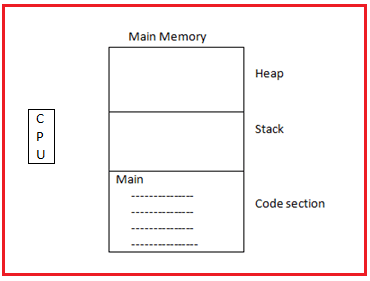As you can see in the above image, there is the main memory and CPU which will execute our programs. As we already discussed in our previous articles, the main memory is divided into three parts i.e. Code Section, Stack, and Heap. Our program code (main function or other functions) reside in the code section.

The program can directly access the Code Section and the Stack section of the Main memory. The program will not directly access the heap. So, the heap memory is external to the program, outside the program and the program doesn’t access it directly.

##### How to access the heap area by the program?

To access the heap area by the program we need a pointer. So, one of the reasons for using a pointer is to access the heap area. So, the program should have a pointer with itself, and with that pointer, we can access anything in the heap area such as an array or any other variables. For a better understanding, please have a look at the below image.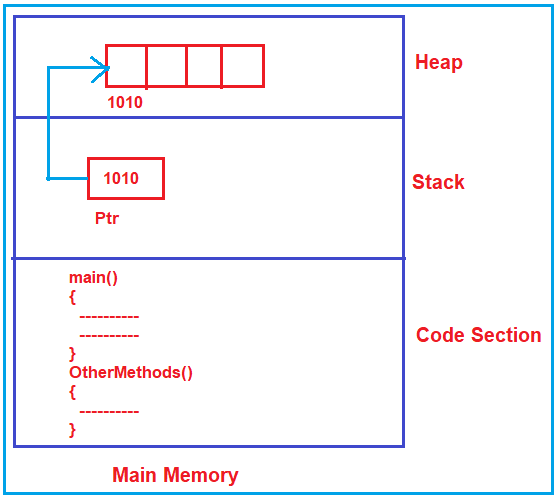Note: Pointer is useful for accessing the resources that are outside of the program i.e. outside of the code section and heap memory.

##### Other Scenarios where we need Pointers:

Suppose on the hard disk we have some files. Then the program cannot access the hard disk files directly because the hard disk is external or files are external to a program. So, for that, it needs a pointer for accessing and the pointer should be a file type so that it can access that file.

In some scenarios, the program may be accessing a keyboard, the program may be accessing a monitor, or maybe accessing the internet or network connection. All these things are external to a program, all these things can only be accessed with the help of pointers.

So, one major use of pointer is accessing the resources which are outside the program. Pointers are used for accessing heap memory, accessing resources, and parameter passing also.

##### How to declare a pointer, how to initialize it, and how to use it in C Language?

Let’s take an example. Please have a look at the below image.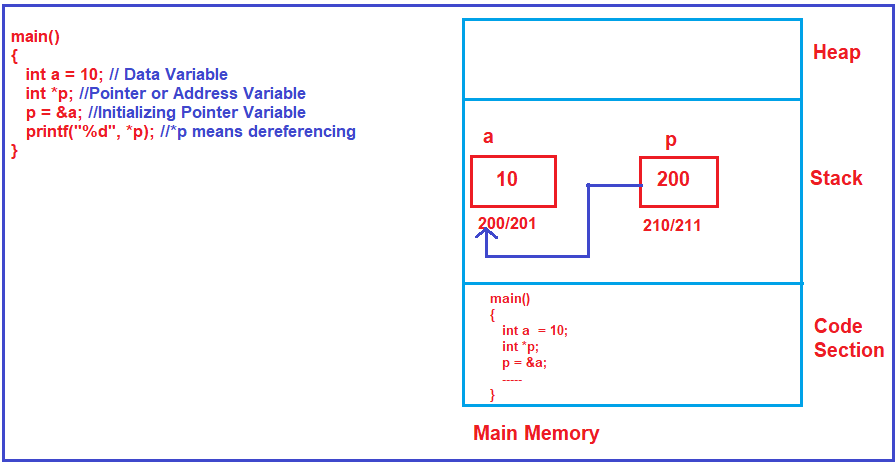As you can see in the above image, here we are declaring a data variable “a” and also initializing it with a value of 10. Pointer variables are also called address variables in C and C++ language. Here, *p is a pointer variable. In our example, both a and *p are going to be created in the Stack area of the Main memory. Then we initialize the pointer variable (p = &a) to address the data variable a. So, the pointer now storing the address of a. * is called dereferencing.

##### How to access heap memory using a pointer?

Whenever we declare any variable whether it is a data variable or pointer variable, it is going to be created inside the Stack frame of the Main memory. So, in the previous example, both the variables are created inside the Stack memory only. Now, the question is how we will get memory in the heap?

##### How to get memory in the heap?

In C language, there is a function called malloc(). When we write malloc(), then only we will get the memory in the heap. In order to use the malloc() function first we need to include the #include<stdlib.h> header file.

While using the malloc() function we need to specify the size i.e. how many bytes of memory we want in the heap. For example, let’s say we want memory for 5 integers in the heap. Then we need to specify the size as shown below. Here the sizeof(int) will give the size of an integer in bytes depending on the compiler and operating system we used. If the compiler takes 2 bytes for an integer then it will allocate 10 bytes in the heap memory. If the compiler takes 4 bytes for an integer then it will allocate 20 bytes in the heap memory.

malloc(5 * sizeof(int));

The malloc function returns a void pointer. So, we need to typecast it to an integer pointer as shown below.
int *p;
p = (int *) malloc(5 * sizeof(int));

In C++, you can do the same using a new operator as shown below.
int *p;
p = new int;

For a better understanding of the memory architectures, please have a look at the following image.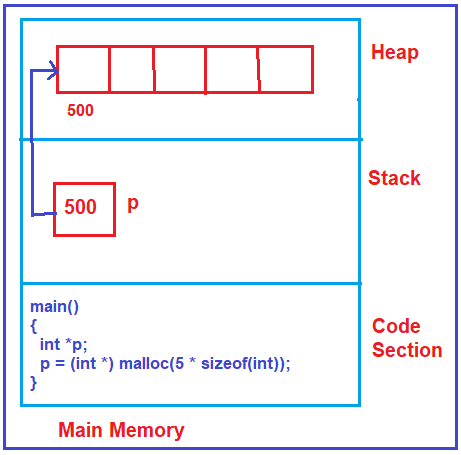I hope you understand the basics of the pointer. Now let us proceed and understand the concept pinter in-depth with more examples.

##### What are Pointers in C?

A Pointer is a derived data type in C that is constructed from the fundamental data type of C Language. A pointer is a variable that holds the address of another variable. A pointer can be defined as it is a memory variable that stores a memory address. It is denoted by the ‘*’ operator.

##### What are the Advantages of using Pointers in C Langauge?
1. Data access: C programming language is a procedure-oriented language i.e. applications are developed by using functions. From one function to another function, when we are required to access the data, a pointer is required.
2. Memory Management: By using pointers only, dynamic memory allocation is possible i.e. we can handle runtime memory management.
3. Database/ Data structures: By using pointers we can handle the data structure more efficiently. Any kind of data structure is required to develop by using pointers only, if data structures are not available then the database is not possible to create.
4. Performance: By using pointers, we can increase the execution speed of the program.
5. By using a pointer, we can access the data which is available outside of the function.
##### Operators used in Pointer:

When we are working with the pointer, we need to use the following two operators.

1. * (Indirection operator or dereference operator or object at location or value at address)

The & is a unary operator. The address-of operator always returns the base address of a variable. The starting cell address of any variable is called the base address.

##### * (Indirection operator):

The Indirection (*) Operator is a unary operator. It is used to create a pointer variable. Indirection Operator always returns the value of an address, i.e. what address we are passing, from that address corresponding value will be retrieved.

##### Declaration of Pointer:

Syntax: Datatype *ptr_name;
Example: int *p;
Here, the * mark before the variable indicates that it is a pointer variable. Indirection operators must be required between datatype and variable name. Space is not mandatory to place in the declaration of the pointer variable.

##### Initialization of Pointer:

Syntax:
Datatype variable;
Datatype *ptr = &variable;

##### Example to Understand How to Create a Pointer in C Language:
```void main()
{
int i;
Int * ptr;
}
```

Here, i is a variable of type integer and it is a value type variable that always holds an integer value. ptr is a variable of type int* and it is an address type variable that always holds an integer variable address.

##### Program:
```#include <stdio.h>
int main()
{
int a;
printf("Enter a Number: ");
scanf("%d", &a);
printf("Entered Value is %d: ", a);
printf("\n");
printf("The Address is %d: ", &a);
return 0;
}
```
###### Output: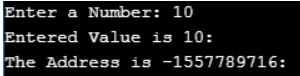##### Example to show the use of Pointers in C Language
```#include<stdio.h>
int main ()
{
int a;
int *ptr;
ptr = &a;
a = 10;
printf ("\n %p %p", &a, ptr);
printf ("\n %d %d", a, *ptr);
*ptr = 20;
printf ("\n %u %u", &a, ptr);
printf ("\n %d %d", a, *ptr);
return 0;
}
```
###### Output: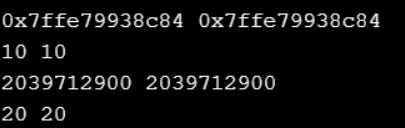On any variable when we are applying address-of operator (&) then always it will collect the base address only i.e. the starting cell location. On address when we are applying indirection operator (*) then it returns the value of that address.

In the above program, ptr holds the address of a (&a) that’s why ptr and & value are the same. *ptr always returns an object i.e. value that’s why ‘*ptr’ and a value are the same.

In implementation when we are printing the address of the variable then go for %x, %p, %u. %lu and %lp format specifiers. %x, %p, %lp will print the address in hexadecimal format, and %u, %lu will print the address in decimal format.

Note: For printing the address we cannot use the %d format specifier because the physical address will be there in hexadecimal format from the range of 0*0000 to 0*ffff, in decimal format 0 to 65535 so this range will not be supported by %d. There is no –ve representation is available in physical address but %d will print –ve data also,

##### Example to show the difference between %p and %x in C Language
```#include<stdio.h>
int main ()
{
int a;
int *ptr;
ptr = &a;
a = 11;
printf ("\n %p %p", &a, ptr);
printf ("\n %d %d", a, *ptr);
*ptr = 22;
printf ("\n %x %x", &a, ptr);
printf ("\n %d %d", a, *ptr);
return 0;
}
```
###### Output: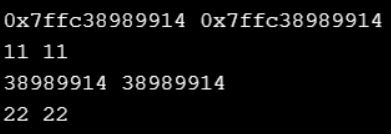##### What will be the output in the below program?
```#include<stdio.h>
int main ()
{
int a, b;
int *ptr;
ptr = &a;
a = 10;
b = 20;
printf ("\n %d %d %d", a, b, *ptr);
*ptr = 30;
ptr = &b;
*ptr = 40;
printf ("\n %d %d %d", a, b, *ptr);
return 10;
}
```
###### Output: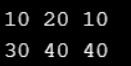Note: In implementation when we need to change the pointer location from one variable to another variable, then by re-assigning the address to the pointer, we can change the pointer location.

##### Data Access mechanism using Pointers in C Language:

Different types of variables are having different types of sizes because internal content is different. Any type of pointer has the same size only because internal content is the address which is common for any type of variable.

Any kind of pointer can hold any kind of variable address but at the time of manipulating the data, we see the difference. In implementation when we need to hold an integer variable address then go for int *, float variable address then go for float * and for char variable address go for char * only.

DOS Operating system doesn’t have any memory management that’s why at run time addresses are limited. On the DOS-based compiler, the size of the pointer is 2 bytes because the address is limited. Windows, Unix, and Linux OS are having proper memory management that’s why the address is not limited (depends on RAM capacity). On a windows-based compiler, the size of the pointer is 4 bytes because addresses are unlimited. On 64-bit OS, when we are using a 64-bit compiler then the size of the pointer is 8 bytes. On 32-bit OS, we are using a 16-bit compiler then the size of the pointer is 2 bytes. On 64-bit OS, a 16-bit compiler is not compatible only 32-bit & 64-bit compilers will work.

Any type of pointer size is 2 bytes only because it maintains the offset address from the range of 0X0000 to 0XFFFF, so for holding this many addresses we require 2 data. Any type of pointer holds single-cell info only i.e. Base address, but data can be present in multiple cells. On address when we are applying indirection operator than its deference to a pointer type, so depending on pointer type given base address (n no. of bytes will be accessed). In C programming language, any type of pointer can hold, any kind of variable address because the address doesn’t maintain any type of information. In implementation when we are manipulating an integer variable then go for an int*, float variable float* and character variable is char* because we can see the difference when we are applying the indirection operator.

##### Pointers Example 1 using C Language:
```#include<stdio.h>
int main ()
{
int a, b;
char *ptr;
ptr = &a;
a = 32767;
b = *ptr;
printf ("%d %d %d", a, b, *ptr);
*ptr = 0;
printf ("\n%d %d %d", a, b, *ptr);
return 0;
}
```
###### Output: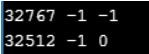On integer variable, when we are applying char pointer, then it can access and manipulate only 1byte data because indirection operator behavior is datatype dependent.

##### Pointers Example 2 using C Language:
```#include<stdio.h>
int main ()
{
int a, b;
char *ptr;
ptr = &a;
a = 543;
b = *ptr;
printf ("%d %d %d", a, b, *ptr);
*ptr = 255;
printf ("\n%d %d %d ", a, b, *ptr);
return 0;
}
```
###### Output: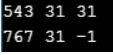All integer variables, when we are applying char ptr then always it will access low order byte data only. When we are working with 2 bytes integers, then the 1st byte is called the low order byte and the 2nd-byte data is called the high order byte.

##### Pointers Example 3 using C Language
```#include<stdio.h>
int main ()
{
int a, b;
char *ptr;
ptr = &a;
a = 511;
b = *ptr;
printf ("\n %d %d %d", a, b, *ptr);
*ptr = 10;
printf ("\n %d %d %d", a, b, *ptr);
return 0;
}
```
###### Output: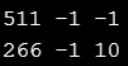Note: we can see this difference in Turbo-c but in the case of GCC and Dev C it will give the compile-time error.